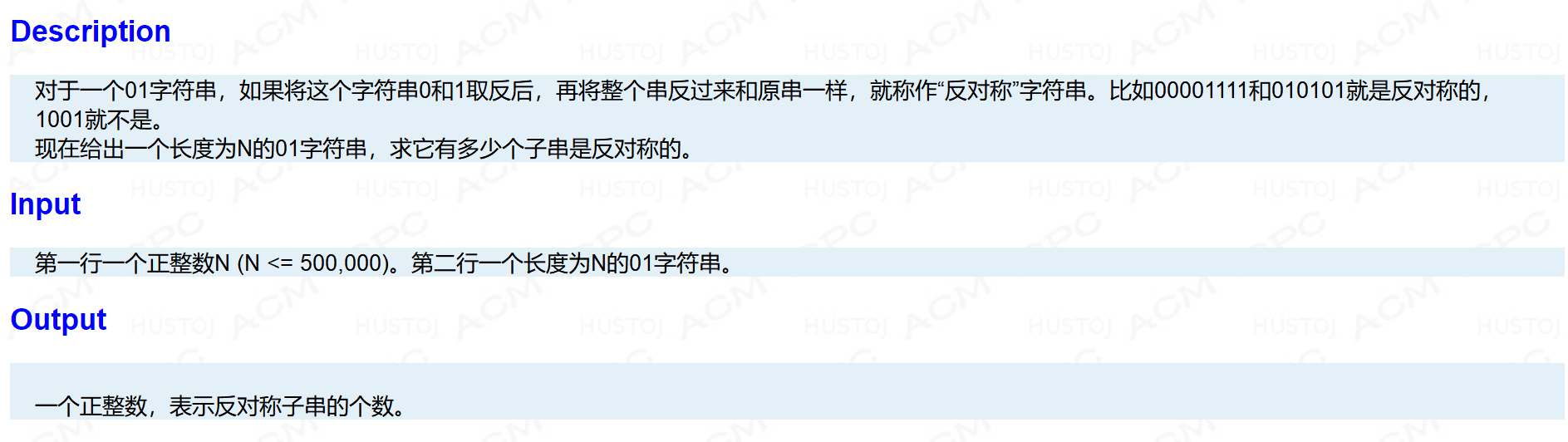# 【BZOJ2084】【洛谷P3501】[POI2010]ANT-Antisymmetry（Manache算法）

题意描述

原题：一句话描述：对于一个0/1序列，求出其中异或意义下回文的子串数量。

我们可以看出，这个其实是一个对于异或意义下的回文子串数量的统计，什么是异或意义下呢？平常，我们对回文的定义是，对于任意$i$,$S[i]=S[n-i+1]$，而我们把相等改为异或操作，那么，当且仅当$1$与$0$相匹配时，返回值为$1$ 也就是 “真”。

那么，我们可以尝试使用Manache算法来解决。当然，编程时，我们并不必真的去把0/1序列转换为数字序列，进行异或操作，这样会给自己增加一波常数（迷），我们构造一个to数组，$to[x]$数组的定义为 对于字符$x$ 我们允许匹配的对应字符，显然，$to['0']='1'$,$to['1']='0'$，特别的$to['\#']='\#'$  $to['\$']='\$'$。（此处'#'与'$'是Manache算法的分隔字符与防止溢出字符，可以自定义）。 对于Manache算法有任何不了解的地方，可以戳!!!这里!!!，又看不懂的地方，也可以联系文文(434935191) 对于代码：1 #include<cstdio> 2 #include<algorithm> 3 #include<cstring> 4 const int maxn = 1000010; 5 typedef unsigned long long ull; 6 char SS1[maxn],S[maxn],to; 7 int n,len[maxn],tot=1; 8 int main() { 9 scanf("%d%s",&n,SS1+1);S='$',S='#';
10     for(register int i=1;i<=n;++i) S[++tot]=SS1[i],S[++tot]='#';
11     to['1']='0',to['0']='1',to['#']='#',to['$']='$';
12     int pos=1,mx=1;ull ans=0;
13     for(register int i=1;i<=tot;i+=2) {
14         len[i]=(i<mx?std::min(mx-i,len[(pos<<1)-i]):1);
15         while(S[i+len[i]]==to[S[i-len[i]]]) len[i]++;
16         if(len[i]+i>mx) {
17             mx=len[i]+i;pos=i;
18         }
19         ans+=len[i]>>1;
20     }
21     printf("%llu\n",ans);
22     return 0;
23 }
View Code

posted @ 2018-07-14 20:07  文文殿下  阅读(199)  评论(0编辑  收藏  举报